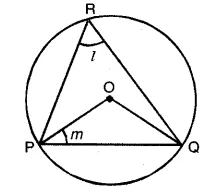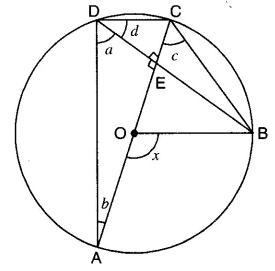# Class 9 – Circles – Important Questions

Circles – Important Questions

1. Prove that if chords of congruent circles subtend equal angles at their centres, then the chords are equal.
2. Draw different pairs of circles. How many points does each pair have in common? What is the maximum number of common points?
3. If two circles intersect at two points, prove that their centres lie on the perpendicular bisector of the common chord.
4. Three girls Reshma, Salma and Mandip are playing a game by standing on a circle of radius 5m drawn in a park. Reshma throws a ball to Salma, Salma to Mandip, Mandip to Reshma. If the distance between Reshma and Salma and between Salma and Mandip is 6m each, what is the distance between Reshma and Mandip?
5. If a line segment joining two points subtends equal angles at two other points lying on the same side of the line containing the line segment, the four points lie on a circle (i.e. they are concyclic).
6. Prove that the quadrilateral formed (if possible) by the internal angle bisectors of any quadrilateral is cyclic.
7. In any triangle ABC, if the angle bisector of ∠A and perpendicular bisector of BC intersect, prove that they intersect on the circumcircle of the triangle ABC.
8. If the non-parallel sides of a trapezium are equal, prove that it is cyclic.
9. ABCD is a cyclic quadrilateral whose diagonals intersect at a point E. If ∠DBC = 70°, ∠BAC is 30°, find ∠BCD. Further, if AB = BC, find ∠ECD.
10. If two circles intersect at two points, prove that their centres lie on the perpendicular bisector of the common chord.
11. Prove that equal chords of a circle subtend equal angles at the centre.
12. If two intersecting chords of a circle make equal angles with the diameter passing through their point of intersection, prove that the chords are equal.
13. In the given figure, O is the centre of the circle. PQ is a chord of the circle and R is any point on the circle. If ∠PRQ = l and ∠OPQ = m, then find l + m.14. A circular park of radius 10 m is situated in a colony. Three students Ashok, Raman and Kanaihya are standing at equal distances on its circumference each having a toy telephone in his hands to talk each other about Honesty, Peace and Discipline.
1. Find the length of the string of each phone.
2. Write the role of discipline in students’ life.
15. In the given figure, AC is a diameter of the circle with centre O. Chord BD is perpendicular to AC. Write down the measures of angles a, b, c and d in terms of x.16. Bisectors of angles A, B and C of triangle ABC intersect its circumcircle at D, E and F respectively. Prove that the angles of the ΔDEF are 90° -∠ A/2 ,90° – ∠ B/2 and 90°- ∠ C/2 respectively.
17. Show that the quadrilateral formed by angle bisectors of a cyclic quadrilateral, is also cyclic.
18. If two equal chords of a circle intersect within a circle, prove that the line segment joining the point of intersection to the centre makes equal angles with the chords.
19. If the non-parallel sides of a trapezium are equal, prove that it is cyclic.
20. Prove that a cyclic parallelogram is a rectangle.